#### Vector spaces

Before defining a vector field, it is helpful to be precise about what is meant by a vector. A vector space (or linear space) is defined as a set,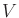, that is closed under two algebraic operations called vector addition and scalar multiplication and satisfies several axioms, which will be given shortly. The vector space used in this section is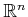, in which the scalars are real numbers, and a vector is represented as a sequence ofreal numbers. Scalar multiplication multiplies each component of the vector by the scalar value. Vector addition forms a new vector by adding each component of two vectors.

A vector spacecan be defined over any field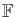(recall the definition from Section 4.4.1). The fieldrepresents the scalars, andrepresents the vectors. The concepts presented below generalize the familiar case of the vector space. In this case,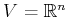and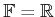. In the definitions that follow, you may make these substitutions, if desired. We will not develop vector spaces that are more general than this; the definitions are nevertheless given in terms ofandto clearly separate scalars from vectors. The vector addition is denoted by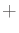, and the scalar multiplication is denoted by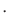. These operations must satisfy the following axioms (a good exercise is to verify these for the case oftreated as a vector space over the field):

1. (Commutative Group Under Vector Addition) The setis a commutative group with respect to vector addition,.
2. (Associativity of Scalar Multiplication) For any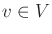and any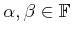,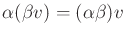.
3. (Distributivity of Scalar Sums) For anyand any,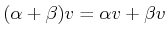.
4. (Distributivity of Vector Sums) For any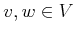and any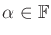,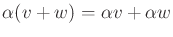.
5. (Scalar Multiplication Identity) For any,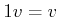for the multiplicative identity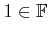.
The first axiom allows vectors to be added in any order. The rest of the axioms require that the scalar multiplication interacts with vectors in the way that we would expect from the familiar vector spaceover.

A basis of a vector spaceis defined as a set,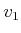,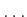,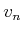, of vectors for which everycan be uniquely written as a linear combination: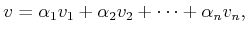(8.7)

for some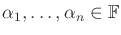. This means that every vector has a unique representation as a linear combination of basis elements. In the case of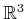, a familiar basis is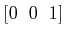,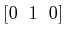, and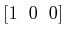. All vectors can be expressed as a linear combination of these three. Remember that a basis is not necessarily unique. From linear algebra, recall that any three linearly independent vectors can be used as a basis for. In general, the basis must only include linearly independent vectors. Even though a basis is not necessarily unique, the number of vectors in a basis is the same for any possible basis over the same vector space. This number,, is called the dimension of the vector space. Thus, we can callan-dimensional vector space over.

Example 8..4 (The Vector SpaceOver)   As indicated already,can be considered as a vector space. A natural basis is the set ofvectors in which, for each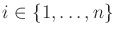, a unit vector is constructed as follows. Let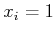and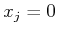for all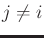. Since there arebasis vectors,is an-dimensional vector space. The basis is not unique. Any set oflinearly independent vectors may be used, which is familiar from linear algebra, in which nonsingular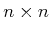matrices are used to transform between them.To illustrate the power of these general vector space definitions, consider the following example.

Example 8..5 (A Vector Space of Functions)   The set of all continuous, real-valued functions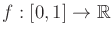, for which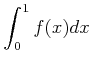(8.8)

is finite, forms a vector space over. It is straightforward to verify that the vector space axioms are satisfied. For example, if two functions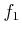and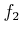are added, the integral remains finite. Furthermore,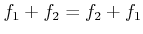, and all of the group axioms are satisfied with respect to addition. Any function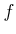that satisfies (8.8) can be multiplied by a scalar in, and the integral remains finite. The axioms that involve scalar multiplication can also be verified.

It turns out that this vector space is infinite-dimensional. One way to see this is to restrict the functions to the set of all those for which the Taylor series exists and converges to the function (these are called analytic functions). Each function can be expressed via a Taylor series as a polynomial that may have an infinite number of terms. The set of all monomials,,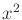,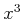, and so on, represents a basis. Every continuous function can be considered as an infinite vector of coefficients; each coefficient is multiplied by one of the monomials to produce the function. This provides a simple example of a function space; with some additional definitions, this leads to a Hilbert space, which is crucial in functional analysis, a subject that characterizes spaces of functions [836,838].The remainder of this chapter considers only finite-dimensional vector spaces over. It is important, however, to keep in mind the basic properties of vector spaces that have been provided.

Steven M LaValle 2020-08-14